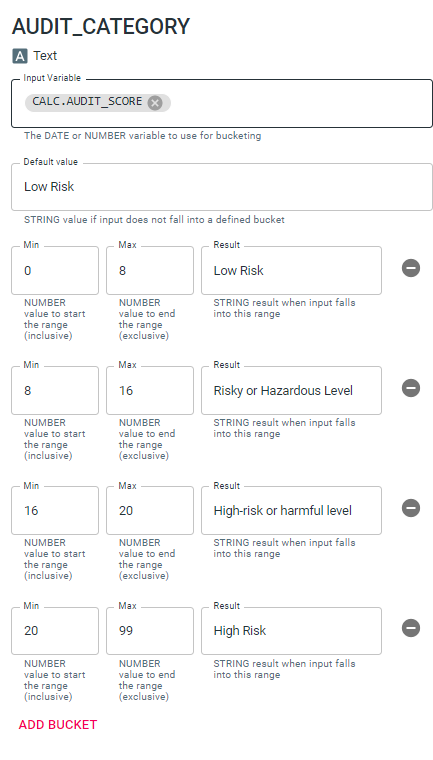Calculations or Calculated Variables provide the means to create calculations based on various data elements. Calculations are most commonly used within a Survey to create a new variable based on survey responses such as 'BAC' or 'AUDIT Score'. However, IMPACT Feedback also provides Calculations in Reports, Messages, and Events.

• Quick Show Condition: Used for the creation of a variable to use in show logic (item condition). Common uses of the Quick Show Condition are to variables used to hide survey questions (or pages) based on the response to a previous question. Use this to create a 'Cannabis Logic' item that will hide subsequent questions about Cannabis if the case responds a certain way to a previous question about Cannabis.

• Conditional Expression: Use this to return one of two possible outputs (either return 'this' or 'that') . The output variable can be another number, a date, text, or True/False.  A conditional expression can be used to return dichotomous output variable (a '1' or a '0') based on a certain survey response. For example, a survey question asks a case how many alcohol drinks they typically consume on each day of the week. The responses that are recorded would provide the number of drinks consumed on each day. However, we may also want to calculate how many days during that week the case consumed at least one drink (total # of days that they drank during that week). In that case a conditional expression could be added for each day of the week that returns a '1' if the case consumed >= 1 drink on that particular day and a '0' if they drank '0' drinks.

• Conditional Bucket:  See table below:

 Conditional Bucket:  A conditional bucket expression works much like a conditional expression except that in this case the input variable (ie: responses to a survey question) can be broken into various buckets with corresponding output. In the example displayed in the image to the right, a conditional bucket expression is used to return a risk category based on the AUDIT Score.  Here, AUDIT scores within defined ranges translate to a particular risk category.• Date Manipulation: Date manipulation adds or subtract from a specified date. For example, a date manipulation calculation could calculate the # of days since the case was created/added to the system.

• Date Number Extract: These calculations are used to extract a numeric component from a date (hour, day, year).

• Formatter: This calculation provides a means for formatting the input variable for display (ie: in a Feedback Report or Message). For example, the 'precision' function can be used to round BAC to the desired number of decimals.

• Math Expression: Math expression calculations provide a robust solution for computing complex math equations. For example, AUDIT Score is calculated by assigning a certain numeric value to each response on the scale.

• Math Function:This calculation type provides the following three options for rounding a numeric value to a whole number.
• Round: Rounds to nearest whole number
• Ceiling: Rounds up to the next whole number (ie: '6.1' becomes '7')
• Floor: Rounds down to the next whole number (ie: '3.7' becomes '3')

• Normative Lookup: Use this to compute normative information typically displayed above normative charts. Normative lookup calculations reference your Organization's norms in order to provide normative percentage or percentile rank to be inserted into the Feedback Report.